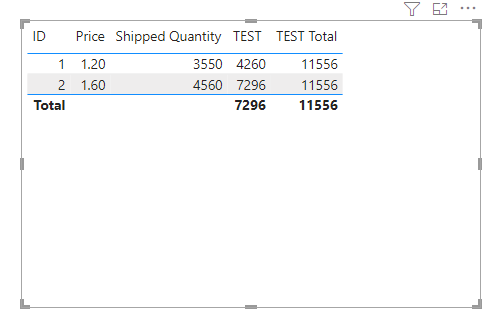cancel
Showing results for
Did you mean:Helper II

## Calculated Measure (Total of measure)

So this may be a simple question...So I am displaying the table on the left and i'm needing to create a calculated measure that multiplies ("Price" x "Shipped Quantity"), There is a measure that calculates the sum(Shipped Quantity) and I have used this in my measure which is giving me incorrect values because that measure takes into account the 2 transactions from the table on the right. I have used the measure SUM(Shipped Quantity) because it is a measure so I am able to use it in my calculation and get a total without having to filter for a specific value.

I want to multiply by 'Shipped Quantity' instead (which is a column, so I cannot just access it directly in my measure without wrapping it in a aggregated function). I have tried using 'Selectedvalue' but I also need to calculate a 2nd measure to get the total of 'TEST' (which is the calculated measure). so this is what i need help with if anybody can suggest something

TEST = Calculate ( [Price] * SelectedValue('Table'[Shipped Quantity] )
This return me the correct value but does not give me a total (is there another way to multiply/use the column in my expression)

TEST Total = SUMX( 'Table',[TEST] )
When doing this calculation to get the total it will return me the total for the table on the right (8520) but I'm wanting the total for the table on the Left (4260)

5 REPLIES 5Helper IV

Hi, I don't know the base table in totality, but you have the following cases:

a) either the above are duplicate shipping lines (the same thing that for whatever reason appears twice in your table). Then the ID= 1 is correct  und you can produce a distinct table that omits duplicate lines and then you are fine with the normal sumx expression.Or you keep the two lines and divide the result by the number of lines of this very ID
b) or the table has not the right ID. This means there should be an ID per line which is the not the one shown, rather some combination of several fields that makes the line unique

If these are really shipping orders with shipping lines, the combination of shipment number and shipping line number should be unique. If not existing you can always number the lines per shipment and create such an ID.

Best regards
ChristianHelper IV

Hi, not entirely sure if this is what you are searching:

TestMeasure = sumx('Table', 'Table'[qty] * 'Table'[price]) will take the row context and give the subtotal of the regarded row (as product of price and qty). If you put this measure as additional column in a table visual in Power BI it gives the subtotal per row. Should be the same with Excel.

Best regards

ChristianHelper II

the problem is when i do the sum its giving me the sum for both trnasactions of the same ID and its incorrectCommunity Support

Hi  @Euro0681 ,

(1) Create new measure

``````TEST = MAX('Table'[Price])*MAX('Table'[Shipped Quantity])

TEST Total = SUMX( ALL('Table'),[TEST] )``````

(2)Final outputIf that's not what you need, provide sample files and expected output.

Best Regards,
Gallen Luo
If this post helps, then please consider Accept it as the solution to help the other members find it more quickly.Helper II

for the total i'm still having the same issue where the total is doubling becuase it's registering both transactions and not giving me the total for the TEST (so my number is inflated)Announcements#### Exclusive opportunity for Women!

Join us for a free, hands-on Microsoft workshop led by women trainers for women where you will learn how to build a Dashboard in a Day!#### Power Platform Conference-Power BI and Fabric Sessions

Join us Oct 1 - 6 in Las Vegas for the Microsoft Power Platform Conference.Top Solution Authors
Top Kudoed Authors
Users online (2,042)